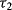# 4.5 LGM with Categorical Outcomes

In Section 2.4 we discussed that the categories of an observed ordered categorical variable y are observed measures of an underlying continuous latent variable y*. For an observed variable with M categories, there would be (M − 1) unknown thresholds,,, . . . , and, that separate the adjacent categories and link the latent continuous y* variable to the observed categorical variable y. When categorical outcomes are involved in a SEM model, rather than the variances/covariances of the observed indicators, the correlations among the latent continuous variables y* are analyzed. As the special correlation matrices do not follow Wishart distribution (Wishart, 1928), special estimation procedures are needed for model estimation. When categorical outcomes are involved, Muthén's robust estimator WLSMV is used for model estimation by default in Mplus (Muthén and Muthén 1998–2010).

For LGM with categorical outcomes, Mplus provides two parameterization approaches: the Delta parameterization and the Theta parameterization (Muthén and Muthén, 1998–2010). When the default Delta parameterization is used, the scale factors of the latent variables () are treated as ...

Get Structural Equation Modeling: Applications Using Mplus now with the O’Reilly learning platform.

O’Reilly members experience books, live events, courses curated by job role, and more from O’Reilly and nearly 200 top publishers.